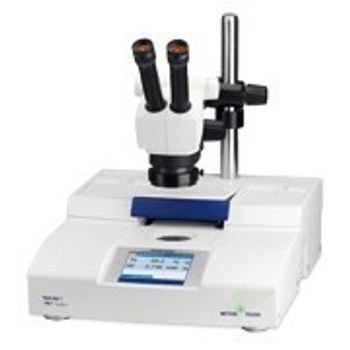Thermal analysis is the study of material's properties when it changes with temperature. Different types of thermal analyzers are available on the specific measurement required. The first thing to consider when purchasing a thermal analyzer is what type of measurement is of interest.

Dynamic Mechanical Analyzers (DMA)(Mechanical Stiffness & Damping Difference)
DMA measures a material's response to temperature, stress, and frequency by applying small deformations in an oscillating manner. There are two main types of DMA, stress control and strain control

Stress Control
A stress controlled instrument ideal for stiff samples, applies a sinusoidal stress (force)to a sample and records the strain in micrometers (displacement), allowing one to determine the complex modules. These instruments can also very frequency, temperature, time and sample deformation geometry.

Strain Control
A strain controlled instrument, ideal for softer samples, applies a sinusoidal strain (displacement) to a sample and records complex modules. These instruments can also very frequency, temperature time and sample deformation geometry.

Differential Thermal Analyzers (DTA)(Temperature Difference)
These instruments measure temperature difference between a reference and a sample by heating both under an identical temperature program. Classically, DTA pre-dated current DSC technology.

Thermogravimetric Analyzers (TGA)(Mass Difference)
TGA instruments measure the change in mass of materials a function of a defined temperature program.

Dielectric Thermal Analyzers (DEA)(Dielectric permittivity & Loss Factor Difference)
DEA measures the maintained electrical current of a sample when there is a change in temperature. The sample is placed in a furnace between metal plates while an alternating voltage is applied across them.

Thermomechanical Analyzers (TMA)(Dimension Difference)
TMA measures the dimensional change (micrometers) in a sample when exposed to a defined temperature program. Various probes are utilized to measure expansion, contraction, penetration, softening of the sample as well as glass transitions.

Simultaneous Thermal Analyzers (STA)
STA is a combined method of measuring weight loss (TGA) and heat flow (DTA/DSC) simultaneously within the same instrument.

The simultaneous measurement of heat flow compliments the loss data by providing information on transitions that do not result in a mass change.

Differential Scanning Calorimetry (DSC)(Heat Flow)
The most commonly used technique in thermal analysis is Differential Scanning Calorimetry (DSC). These analyzers are designed to measure the physical and chemical transitions that take place in materials when exposed to a defined temperature program.

There are two different classes of DSC analyzers: The Heat Flux DSC and the Power Compensated DSC.

Heat Flux DSC
The heat flux DSC uses a common heat flux plate in which there is a sample and reference positions. A common furnace heats both the sample and reference through the desired temperature profile. Temperature sensors under each position monitor and record the differential temperature. This data is converted  into energy units (milliwatts) by the computer and this is the calorimetric heat flow signal

Power Compensated DSC
Power compensated DSC uses independent furnaces and sensors for both the sample and the reference positions. As sample transitions occur, the instrument responds by wither applying or removing power to keep both furnaces equal. This power in milliwatts is the calorimetric heat flow signal.